﻿ An IBM-2 Calculation of E2/M1 Multipole Mixing Ratios of Transitions in <SUP>90-96</SUP>Sr

### An IBM-2 Calculation of E2/M1 Multipole Mixing Ratios of Transitions in 90-96Sr

Saad Naji Abood, Laith Ahmed Najim

International Journal of Physics

## An IBM-2 Calculation of E2/M1 Multipole Mixing Ratios of Transitions in 90-96Sr

Saad Naji Abood1, Laith Ahmed Najim2,1Department of Physics, College of Science, AL-Nahrain University, Baghdad, IRAQ

2Department of Physics, College of Science, Mosul University, Mosul, IRAQ

### Abstract

The interacting boson model is applied to the even strontium isotopes, 90-96Sr. Excitation energies, electromagnetic transition strengths, quadrupole and δ(E2/M1) multipole mixing ratios have been described systematically. It is seen that the properties of low-lying levels in these isotopes, for which the comparison between experiment and theory is possible, can be epistemologically satisfied by the Interacting Boson Model-2 (IBM-2).

• Saad Naji Abood, Laith Ahmed Najim. An IBM-2 Calculation of E2/M1 Multipole Mixing Ratios of Transitions in 90-96Sr. International Journal of Physics. Vol. 4, No. 1, 2016, pp 5-10. http://pubs.sciepub.com/ijp/4/1/2
• Abood, Saad Naji, and Laith Ahmed Najim. "An IBM-2 Calculation of E2/M1 Multipole Mixing Ratios of Transitions in 90-96Sr." International Journal of Physics 4.1 (2016): 5-10.
• Abood, S. N. , & Najim, L. A. (2016). An IBM-2 Calculation of E2/M1 Multipole Mixing Ratios of Transitions in 90-96Sr. International Journal of Physics, 4(1), 5-10.
• Abood, Saad Naji, and Laith Ahmed Najim. "An IBM-2 Calculation of E2/M1 Multipole Mixing Ratios of Transitions in 90-96Sr." International Journal of Physics 4, no. 1 (2016): 5-10.

 Import into BibTeX Import into EndNote Import into RefMan Import into RefWorks

### 1. Introduction

The neutron-proton interaction is known to play a dominant role in quadrupole correlations in nuclei. As a consequence, the excitation energies of collective quadrupole excitations in nuclei near a closed shell are strongly dependent on the number of nucleons outside the closed shell.

The 90-92-94-96Sr isotopes (Z=38), with neutron number varies from 52 to 58 are known to exhibit have Nπ =1 and Nv varies from 1 to 4. Lie in the transitional region that occurred at the lower limit of the range of deformed nuclei.

The interacting boson approximation has been quite successful at describing the collective properties of several medium nuclei. The interacting boson model (IBM) presented by Arima and Iachello [1, 2, 3, 4] and Casten  has become widely accepted as a tractable theoretical scheme of correlating, describing and predicting low-energy collective properties of complex nuclei. In this model, the low-energy states of even−even nuclei are described in terms of interactions between s (J=0) and d (J=2) bosons. The corresponding Hamiltonian is diagonalized in this boson space by employing somewhat powerful and effective group theory methods.

The outline of the remaining part of this paper is as follows: It starts from an approximate IBM-2 formulation for the Hamiltonian, reviewing the theoretical background of the study. Previous experimental and theoretical data are compared with estimated values when the general features of Sr isotopes in the range A= 90-96.

In recent years many works have had been done on the structure of Sr nucleus. In this work we extended the available systematic IBM-2 calculations of Strontium region.

### 2. The Interacting Boson Model

It is proposed that the change from spherical to deformed structure is related to an exceptionally strong neutron-proton interaction. It is also suggested that the neutron-proton effective interactions have a deformation producing tendency, while the neutron-neutron and proton-proton interactions are of spheriphying nature [6, 7]. Within the region of medium-heavy and heavy nuclei, a large of nuclei exhibit properties that are neither close to an harmonic quadrupole vibrational spectra nor to deformed rotors . While defining such nuclei in a geometric description , these phenomena will have a standard description that is given in terms of nuclear triaxiality , going from rigid triaxial shapes to softer potential energy surfaces. In the first version of the interacting boson model (IBM-1) , no distinction is made between proton and neutron variables while describing triaxiality explicitly. This can be done by introducing the cubic terms in the boson operators . This is a contrast to the recent work of Dieperink and Bijker [13, 14] which showed triaxiality occurs in particular dynamic symmetries of the IBM-2 that distinguish between protons and neutrons.

In the present work, the IBM-2 states that the low lying collective state of even-even nuclei can be described by the interaction of s and dbosons, carrying angular momentum l = 0 and l = 2, respectively.

The IBM-2 Hamiltonian is written :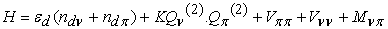(1)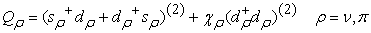(2)

where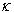is the quadrupole-quadrupole strength and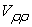is the boson-boson interaction, which is given by the equation: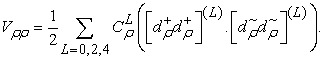and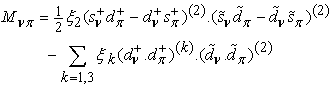(3)

The Majorana term Mπν shifts the states with mixed proton-neutron symmetry with respect to the totally symmetric ones. Since little experimental information is known about such states with mixed symmetry , we did not attempt to fit the parameters appearing in equation (3) , but rather that took constant values for all Sr isotopes.

The general one-body E2 transition operator in the IBM-2 is :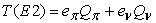(4)

Where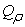is in the form of equation (2). For simplicity, the χρ has the same value as in the Hamiltonian. This is also suggested by the single j-shell microscopy. In general, the E2 transition results are not sensitive to the choice of eν and eπ , whether eν = eπ or not.

The B(E2) strength for E2 transitions is given by: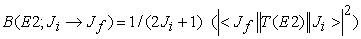(5)

In the IBM-2, the M1 transition operator up to the one-body term is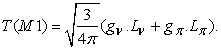The gν and gπ are the boson g-factors in nuclear magneton units, that depend on the nuclear configuration. They should be different of different nuclei. Where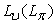is the neutron and (proton) angular momentum operator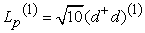.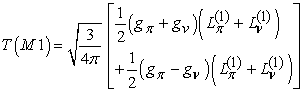(6)

The B(M1) strength for M1 transitions is given by: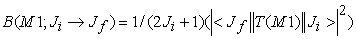(7)

Instead of evaluate the E2 and M1 matrix elements for the Sr isotopes under study which are essential in the theoretical mixing ratio calculations, it is possible to determine these ratios in an analytical form. The calculated E2/M1 mixing ratio: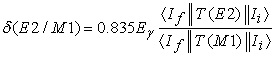(8)

Where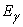is called the transition energy and in MeV and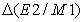is in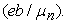### 3. Results and Discussion

3.1. IBM-2 Hamiltonian Parameters

The computer program NPBOS  was used to make the Hamiltonian diagonal. In principle, all parameters can be varied independently in fitting the energy spectrum of one nucleus. However, in order to reduce the number of free parameters and in agreement with microscopic calculations of Guilu et al., , only ε and κ are vary as a function to both of Nπ and Nv i.e. ε = ε( Nπ, Nv ) and κ = κ( Nπ, Nv) are allowed . The other parameters depend only on Nπ or Nv, i.e. χπ = χπ( Nπ), χν = χν= ( Nv), CLπ= CLπ( Nπ) and CLν= CLv ( Nv). Thus, in isotopes chain, χπ is kept constant , whereas for two isotonic Sr isotopes, χν, CLπ and CLν are kept constant (see Table 1).

In principle, the boson numbers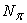and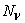can be treated as parameters, but they are taken to be fixed here, counted as half the number of particles and holes outside of the nearest closed shell. We have considered the Z = 28 and 50 as closed shell for this calculation as large quadruple deformations were measured for Z = N= 40 nuclei  and therefore no N = 40 spherical sub-shell closure exists in this region.

The isotopes 90−96Sr have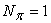, andvaries from 2 to 5, while the parameters,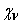, and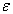were treated as free parameters and their values were estimated by fitting to the measured level energies. This procedure was made by selecting the “traditional” values of the parameters and then allowing one parameter to vary while keeping the others constant until a best fit was obtained. This was carried out iteratively until an overall fit was achieved. The best fit values for the Hamiltonian parameters are given in Table 1.

#### Table 1. IBM-2 Hamiltonian parameters , all parameters in MeV units except χπ and χν are dimensionless

3.2. Energy Levels

Using the parameters in Table 1, the estimated low-lying energy levels are shown in Table 2, along with experimental energy levels. As can be seen, the agreement between experiment and IBM-2 results is quite good and the general features are reproduced well. The discrepancy between IBM-2 results and experiment for high spin states is observed. But one must be careful in comparing theory with experiment, since all calculated states have a collective nature, whereas some of the experimental states may have a particle-like structure. Behavior of the ratio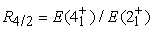of the energies for the first 4+ and 2+ states are good criteria for the shape transition. The value of R4/2 ratio has the calculated values which change from 3 to 2 by increasing the neutron number, R4/2 remains greater than 2 or all the isotopes. It implies that this structure seems to be varying from deformed rotor to very near harmonic vibrator (SU(3) to SU(5)).

In our calculation, the nuclei are nearly spherical with vary small oblate deformation. This is consistent with the work of Hirata et al., . At the beginning of the shell from N = 50, the nucleus is close to the vibrational limit. As the neutron number increases, the nucleus is slowly becoming gamma unstable or O(6) limit.

IBM-2 provides a good agreement with the available experimental data for the energy levels and transition probabilities. It shows a strong evidence of transition from SU(3) to SU(5) symmetry when neutron number increases from N = 52 to 58.

#### Table 2. Low Lying Energy Levels for 90-96Sr (in MeV unit)

In this work the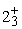state in these isotopes are well described by the lowest mixed symmetry state in the vibrational limit of IBM-2. The validity of this limit is related to the proximity to the closed neutron shell at N =50 and a more detailed calculation would be necessary for large N allowing departures from the vibrational limit. One should also allow admixture between states of full symmetry and mixed symmetry, i.e., states of different F-spin , but both of these generalizations introduce many unknown interaction parameters.

3.3. Electric Quadrupole Transition Probability B(E2)

In order to find the value of the effective charge we have fitted the calculated absolute strengths (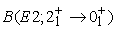) the transitions ground state band to the experimental ones. The values of the boson effective charges for all isotopes, following the work of Subber et al.,  were determined by the experimental, effective charges were obtained that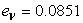e.b and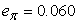e.b. Table 3 given the electric transition probability. The relative B(E2) values are proportional to these effective charges.

#### Table 3. Electric transition probability for Sr90-96 in e2b2units

Theand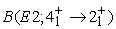values decrease as neutron number increases toward the middle of the shell as the value of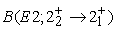has small value because contain mixtures of M1 and The calculated B(E2) value of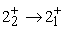transition is between the error limit. Fortransition, the difference between the experimental and theoretical values is very small.

The value of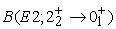is small because there are not deformed nuclei.

However the calculated values in Table 3 are in agreement with the experimental results, there are some difference between the B(E2) values of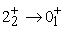transition because there is not enough data and certain result for this transition. The other experimental B(E2) values of some transitions does not exist.

The quadrupole moment for first excited state in Sr isotopes is very well described. The quadrupole moment of the first excited state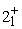is also decreasing toward as neutron number increases. As mentioned above, the calculated values of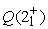indicated this nucleus has oblate shape in first excited states.

3.4. Magnetic Transition Probability B(M1)

To evaluate the magnetic transition probability, the work depend on the Eqs.6 and 7, and determine the values of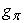and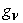. It is interesting to note that the matrix element is approximately proportional to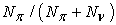and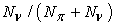, respectively , and directly to the number of active proton and neutron bosons. This leads to a approximate expression :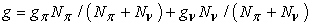(9)

and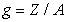, where Z is the atomic number, and A is the mass number.

Therefore the values of g-factor are given as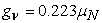and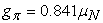. Table 4 gives the values of B(M1) for some transitions, there is a very little experimental data to compare with IBM-2 results.

#### Table 4. Magnetic transition probability for 90-96Sr in μN2units

1. The transitions between low-lying collective states (e.g.,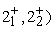are relatively weak since the arise from antisymmetric component in the wave functions introduced by F-spin breaking in the Hamiltonian.

2. Strong transitions connecting a symmetric states,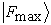with one proton-neutron boson mixed symmetry (e.g.,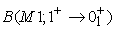) .

3. The magnitude of M1 values increases with increasing spin for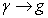and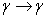transitions.

3.5. Mixing Ratio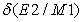Evaluating the mixing ratiofor Sr isotopes, depend on the equation (8), Table 5, shows the variation of 6 for the group of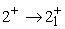transitions and it is seen that both the magnitude and sign ofare correctly obtained for the three transitions a summary of the results where the experimental data have sufficient precision for a useful comparison and also when there is no ambiguity in the nature of the levels. (At higher energies where the level density is great the order of the experimental levels may differ from the calculated order). These results exhibit disagreement in some cases, with one case showing disagreement in sign. However, it is a ratio between very small quantities and any change in the dominator that will have a great influence on the ratio. The large calculated value for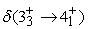is not due to a dominate E2 transition, but may be under the effect of very small M1 component in the transition.

The reduced E2 and M1 matrix elements have been evaluated for a selection of transitions in tungsten isotopes (A = 90,92,94,96); their dependence on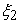is striking. A sudden change in sign is sometimes observed in M1; it occurs when the E2 matrix element is small. It may be attributed to a very low value of the E2 reduced matrix element; even though the program has an arbitrary sign choice, the sign is consistent for all results within a calculation, and the sign of the ratio of the matrix elements which determine the sign of the multipole mixing ratio is not arbitrary.

The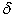-mixing ratios were calculated for some selected transitions in 90-96Sr Tables 5 show comparisons with experimental results. The parameters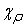,and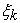, and were kept at their best-fit values and the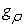were fine-tuned in order to fit the experimental data. In particular,was found to be very sensitive to the-mixing ratios. Good agreement was achieved with the set of parameters (see Table 1). We are now in a position to use these Hamiltonian parameters confidently to o calculate-mixing ratios for any transition in these isotopes.

The variations in sign of the E2/M1 mixing ratios from nucleus to nucleus for the same class transitions and within a given nucleus for transitions from different spin states suggest that a microscopic approach is needed to explain the data theoretically. For that reason, we did not take into consideration the sign of mixing ratios. Sign convention of mixing ratios had explained in detail by J. Lang et al., .

### 4. Conclusions

In this work we have carried out an analysis for the even mass Sr isotopes based on the IBM-2. The boson core parameters have been obtained and the main results for energy levels and quadrupole transition probabilities agree very well with experiment. In general, good agreement was obtained when compared with experiment. The boson-boson interaction parameters were fixed by the calculations on the boson core nuclei. The results indicate that the energy spectra of all different quasibands of the even-even Sr isotopes can be reproduced quite well. It is noticed, however, that the results of B(E2) calculations for even-even Sr nuclei were in better agreement with the existing experimental data. The best fit values for the Hamiltonian parameters for even-even Sr isotopes are given in Table 1, and the calculated energy values which are compared with the experimental data are given in Table 1, Sr isotopes. The agreement is good for member of ground state, ground and beta bands.

The calculated values in this study show that the transitions connect the levels with the same parity and the E2 transitions are predominant. A sensitive test of our projection is provided by comparing calculated B(E2) values with experimental predictions. The agreement between the values obtained in this analysis and the experimental results is good for ground state band, hoping that if the other parameters are normalized by means of this projection it can be considerably improved for gamma band and beta band for further work.

In this work, the mixing ratioof transitions linking the gamma and ground state bands have been examined. The transitions which link low spin states and that obtained in the present work are in good agreement and show a little bit irregularities.

### References

  F. Iachello and A. Arima, The Interacting Boson Model, (Camb. Univ. Press, (1987).In article View Article  A. Arima and F. Iachello, Ann. Phys. NY, 99 (1976) 253.In article View Article  A. Arima and F. Iachello, Ann. Phys. NY, 111 (1978) 201.In article View Article  A. Arima and F. Iachello, Ann. Phys. NY, 123 (1979) 468.In article View Article  R. F. Casten, Nucl. Phys., A 347 (1980) 173.In article View Article  C. K. Nair, A. Ansari and L. Satpathy, Phys. Lett., 71B, (1977), 257-261.In article View Article  A. Sevrin, K. Heyde, and J. Jolie, Phys. Rev. C 36, (1987) 2631-2638.In article View Article  PubMed  M. Sambataro, O. Scholten, A. E. L.Dieperink and G. Piccitto, Nucl. Phys. A423 (19984) 333.In article  A. Bohr and B. Mottelson, “Nuclear Structure', Vol. II (Benjamin), New York, (1969).In article  O. Schoten, A. Arima and F. Iachello, Interacting Ann. Phys. (N.Y.), 99 (1976) 468-473.In article  K. Hayde, P. Van Isacker, M. Waroquier and J. Moreau, Phys. Rev., C 29, (1984), 1420-1426.In article View Article  J. Y. Zhang, R. F. Casten, and N. V. Zamfir, Phys. Lett. B 407, (1997) 201-206.In article View Article  A. E. L. Dieperink and R. Bijker, Phys. Lett., 116B, (1982), 77-81.In article View Article  A. E. L. Dieperink, Progress in Particle and Nuclear Physics, edited by D. Wilkinson, Plenum, New York, 9, (1983).In article  A. Arima, T. Otsuka, F. Iachello, T. Talmi, Phys.Lett., 66B, (1977), 205-209.In article View Article  T. Tagziria, M. Elahrash, W. D. Hamilton, M. Finger, J. John, and V. N. Pavlov, J. Phys. G: Nucl. Part. Phys., 16 (1990) 1323-1328.In article View Article  Van Isacker and G. Puddu, Nucl. Phys A348 (1980) 125.In article View Article  Y. X. Liu, G. L. Long and H. Z. Sun, J. Phys. G: Nucl. Part. Phys., 17 (1991) 877-880.In article View Article  T. Otsuka and N. Yoshida, the IBA-2 computer program NPBOS, University of Tokyo (1985), and Japan Atomic Energy Research Institute Report,(1985) JAERI-M85-094.In article  Long Gulilu, Zhang Jinyu and Tian Lin, Commun. Theor. Phys. 29 (1998) 249.In article View Article  D. Hirata, H. Taki, I. Tanihata and P. Ring, Phys. Lett. B 314 (1993) 168.In article View Article  ENSDF, http:// www.nndc.bnl.gov/ensdf (Nuclear data sheet) (2011).In article  A. R. Subber, P. Park, W. D. Hamilton, K. Kumar, K. Schreckenbash and G. Colvin, J. Phys. G: Nucl. Phys. 12 (1986) 881In article View Article  H. Mach et al., Nucl. Phys. A 523 (1991) 197.In article View Article  C. M. Baglin, Nucl. Data Sheets 66 (1962) 347.In article View Article  J. K. Tuli. Nucl. Data Sheets 66 (1992) 1.In article View Article  J. Lang, K. Kumar and J. H. Hamilton, Rev. Mod. Phys. Vol.54 No. 1 (1982).In article